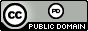시간 제한 메모리 제한 제출 정답 맞은 사람 정답 비율
1 초 1024 MB 7 5 4 66.667%

## 문제

Coach is fed up with sports rankings -- he thinks those who make up these bogus orderings are just nuts.  In Coach's opinion changes in rankings should be evidence-based only.  For example, suppose the $4$th place team plays the $1$st place team and loses.  Why should the rankings be altered? The "worse" team lost to the "better" team, so nothing should change in the rankings.  Put another way, there's no evidence that the ordering should change so why change it?  The only time you change something is if, say, the $4$th place team beats the $1$st place team.  NOW you have evidence that the rankings should change!  Specifically, the $1$st place team should be put directly below the $4$th place team (we now have evidence that backs this up) and the teams in $2$nd through $4$th place should each move up one.  The result is that the former $1$st place team is now in $4$th, one position below the team that beat it, the former $4$th place team now in $3$rd.  Note that the relative positions of the teams now in $1$st to $3$rd place do not change -- there was no evidence that they should.

To generalize this process, assume the team in position $n$ beats the team in position $m$.  If $n < m$ then there should be no change in the rankings; if $n > m$ then all teams in positions $m+1, m+2, \ldots, n$ should move up one position and the former team in position $m$ should be moved to position $n$.

For example, assume there are $5$ teams initially ranked in the order T$1$ (best), T$2$, T$3$, T$4$, T$5$ (worst).  Suppose T$4$ beats T$1$.  Then as described above the new rankings should become T$2$, T$3$, T$4$, T$1$, T$5$.  Now in the next game played let's say T$3$ beats T$1$. After this the rankings should not change -- the better ranked team beat the worse ranked team.  If in the next game T$5$ beats T$3$ the new rankings would be T$2$, T$4$, T$1$, T$5$, T$3$, and so on.

Coach was all set to write a program to implement this scheme but then he heard about ties in the English Premier League.  The last we saw him he was standing motionless, staring out of his window.  We guess it's up to you to write the program.

## 입력

Input begins with a line containing two positive integers $n$ $m$ ($n, m \leq 100$) indicating the number of teams and the number of games played.  Team names are $\mbox{T}1, \mbox{T}2, \ldots, \mbox{T}n$ and initially each team $\mbox{T}i$ is in position $i$ in the rankings (i.e., team $\mbox{T}1$ is in $1$st place and team $\mbox{T}n$ is in last place).  Following the first line are $m$ lines detailing a set of games in chronological order.  Each of these lines has the form $\mbox{T}i$ $\mbox{T}j$ ($1 \leq i,j \leq n, i \neq j$) indicating that team $\mbox{T}i$ beat team $\mbox{T}j$.

## 출력

Output a single line listing the final ranking of the teams. Separate team names with single spaces.

## 예제 입력 1

5 3
T4 T1
T3 T1
T5 T3


## 예제 출력 1

T2 T4 T1 T5 T3


## 예제 입력 2

8 4
T4 T1
T1 T2
T2 T3
T3 T4


## 예제 출력 2

T1 T2 T3 T4 T5 T6 T7 T8


## 출처• 문제를 만든 사람: John Bonomo